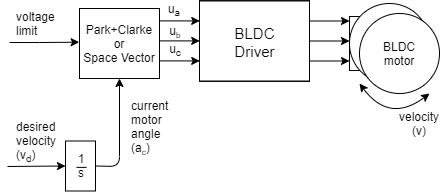# Velocity open-loop control

This control loop allows you to spin your BLDC motor with desired velocity without using position sensor. This mode is enabled by:

``````// set velocity control open-loop mode
motor.controller = MotionControlType::velocity_openloop;
``````You can test this algorithm by running the examples in `motion_control/openloop_motor_control/` folder.

This control algorithm is very simple. User can set the target velocity it wants to achieve vd, the algorithm is going to integrate it in time to find out what is the angle it needs to set to the motor ac in order to achieve it. Then the maximal allowed voltage `motor.voltage_limit` is going to be applied in the direction of the ac using `SinePWM` or `SpaceVectorPWM` modulation.

This is the simplified version of the calculation of the next angle to set to the motor:

``````next_angle = past_angle + target_velocity*d_time;
``````

You need to know the `target_velocity`, sample time `d_time` and past value of the angle `past_angle` you have set to the motor.

## Configuration

``````// choose FOC modulation (optional) - default SinePWM
motor.foc_modulation = FOCModulationType::SpaceVectorPWM;

// limiting voltage
motor.voltage_limit = 3;   // Volts
// or current  - if phase resistance provided
motor.current_limit = 0.5 // Amps
``````

This type of motion control is highly inefficient therefore try not to use to high value for `motor.voltage_limit`. We suggest you to provide the motor class with the `phase_resistance` value and set the `motor.current_limit` instead the voltage limit. This current might be surpassed but at least you will know an approximate current your motor is drawing. You can calculate the current the motor is going to be producing by checking the motor resistance `phase_resistance` and evaluating:

``````voltage_limit = current_limit * phase_resistance; // Amps
``````

Also, you can change the voltage/current limit in real-time if you need this kind of behavior in your application.

## Velocity open-loop control example

Here is one basic example of the velocity open-loop control with the complete configuration. The program will set the target velocity of `2 RAD/s` and maintain it, and the user cna change the target velocity using serial terminal.

``````// Open loop motor control example
#include <SimpleFOC.h>

// BLDC motor & driver instance
// BLDCMotor( pp number , phase resistance)
BLDCMotor motor = BLDCMotor(11 , 12.5);
BLDCDriver3PWM driver = BLDCDriver3PWM(9, 5, 6, 8);

//target variable
float target_velocity = 2; // rad/s

// instantiate the commander
Commander command = Commander(Serial);
void doTarget(char* cmd) { command.variable(&target_velocity, cmd); }

void setup() {

// driver config
// power supply voltage [V]
driver.voltage_power_supply = 12;
driver.init();
// link the motor and the driver

// limiting motor current (provided resistance)
motor.current_limit = 0.5;   // [Amps]

// open loop control config
motor.controller = MotionControlType::velocity_openloop;

// init motor hardware
motor.init();

Serial.begin(115200);
_delay(1000);
}

void loop() {

// open loop velocity movement
// using motor.current_limit and motor.velocity_limit
motor.move(target_velocity);

// user communication
command.run();
}

``````